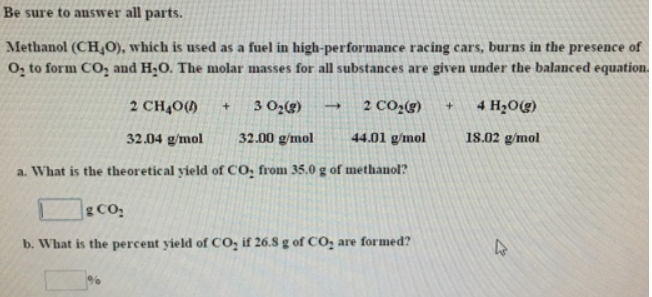# Problem: Be sure to answer all parts.Methanol (CH4O), which is used as a fuel in high-performance racing cars, burns in the presence of O2 to form CO2 and H2O. The molar masses for all substances are given under the balanced equation. 2CH4O (l)       +   3O 2 (g)         →   2CO 2 (g)        +  4H 2O(g) 32.04 g/mol       32.00 g/mol       44.01 g/mol        18.02 g/mol a. What is the theoretical yield of CO 2 from 35.0 g of methanol? b. What is the percent yield of CO 2 if 26.8 g of CO 2 are formed?

###### FREE Expert Solution
83% (442 ratings)###### Problem Details

Be sure to answer all parts.

Methanol (CH4O), which is used as a fuel in high-performance racing cars, burns in the presence of O2 to form CO2 and H2O. The molar masses for all substances are given under the balanced equation.

2CH4O (l)       +   3O 2 (g)         →   2CO 2 (g)        +  4H 2O(g)

32.04 g/mol       32.00 g/mol       44.01 g/mol        18.02 g/mol

a. What is the theoretical yield of CO 2 from 35.0 g of methanol?

b. What is the percent yield of CO 2 if 26.8 g of CO 2 are formed?